Next: 2.1.2 Quantum Confinement Energies Up: 2.1 Characteristic Energies Previous: 2.1 Characteristic Energies

## 2.1.1 Electron Electron Interaction

An entirely classical model for electron-electron interaction is based on the electrostatic    capacitive charging energy. The interaction arises from the fact, that for every additional charge dq which is transported to a conductor, work has to be done against the field of already present charges residing on the conductor. Charging a capacitor with a charge q requires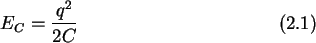energy, where C is the  total capacitance of the capacitor. A first simple model for an island is a conducting sphere which has a  self-capacitance of (see Appendix A.1)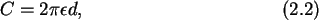where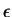is the permittivity of the surrounding dielectric material and d is the diameter of the sphere. Inserting (2.2) in (2.1) yields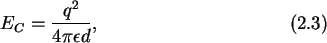an indirect proportionality to the size of the particle. Fig. 2.1 to Fig. 2.3 compare this electrostatic energy to the other characteristic energies involved in charging an island with a small number of electrons. For the sake of simplicity, the relative permittivity,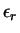, was assumed to be 10. (For relative permittivities of different materials see the Material Properties Table.) A more accurate model for the capacitance of an island is the capacitance of two or more spheres in a line. A calculation for characteristic spatial dimensions shows an increase of about 15% of the total capacitance. See Appendix A for a detailed outline of the calculations, numerical results, and the description of an algorithm to calculate the capacitance of an arbitrary arrangement of spheres. A collection of practical analytical capacitance formulas is given in Table 2.1.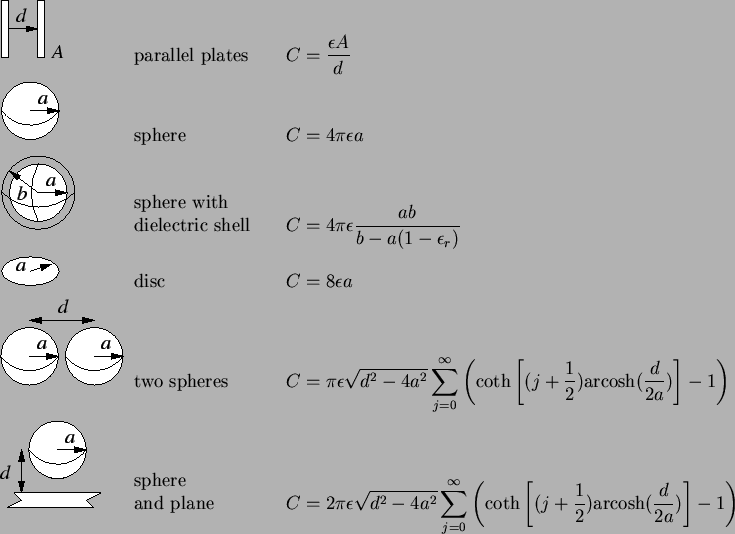For a system of N conductors the charge on one conductor may be written as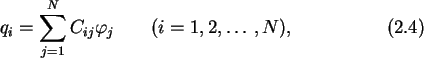where the Cij denote the  elements of the  capacitance matrix. The diagonal elements of the capacitance matrix Cii are the total capacitances of the conductors i and the off-diagonal elements Cij are the negative capacitances between conductor i and j. The electrostatic charging energy is in analogy to (2.1)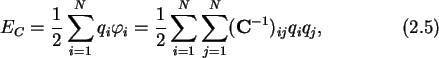where C-1 is the  inverse of the capacitance matrix.

Systems with sufficiently small islands are not adequately described with this model. They exhibit a second electron-electron interaction energy, namely the  change in   Fermi energy, when charged with a single electron. One must distinguish between metals and semiconductors, because of their greatly different free  carrier concentrations and the presence of a band gap in the case of  semiconductors.  Metals have typically a carrier concentration of severaland undoped Si about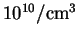at room temperature (see Material Properties Table). The low intrinsic carrier concentration for semiconductors is in reality not achievable, because of the ubiquitous impurities which are ionized at room temperature. These impurities supply free charge carriers and may lift the concentration, in the case of Si, to around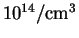, still many orders of magnitude smaller than for metals. Clearly, doping increases the carrier concentration considerably, and can lead in the case of degenerated semiconductors to metal like behavior. We are considering only undoped semiconductors to emphasize the difference to metals.

The dependence of the Fermi energy EF on carrier concentration n is derived in Appendix B (see also ). For metals it is given by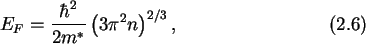and for semiconductors it is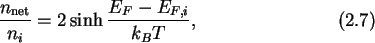where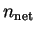is the net carrier concentration defined as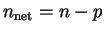. Fig. 2.1 compares the change in the Fermi energy for the addition of one, two, and three electrons in Si to the electrostatic charging energy.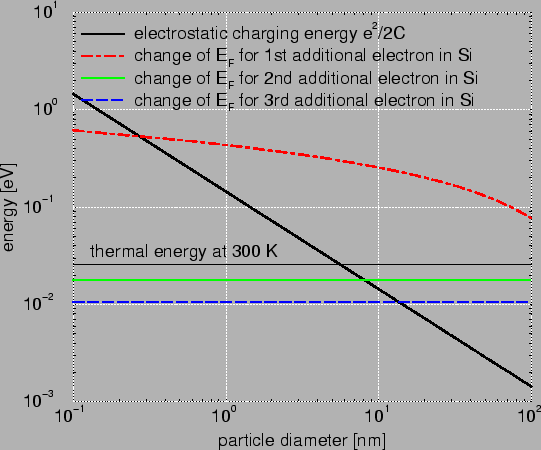The first electron causes the biggest change in Fermi energy. The second and third electron contribute a change smaller than the thermal energy for room temperature. Hence, for single-electron devices one will try to build structures with few free carriers, to achieve a high change in energy when charging an island.

Fig. 2.2 compares Si with Al. It is clearly visible that the change is much bigger in Si. This is caused by two reasons. First, the difference in free carrier concentration. In metals much more carriers are available and therefore the addition of one electron has not such a big impact anymore. Second in metals an additional electron finds a place slightly above the Fermi level. In semiconductors due to the energy gap the electron needs to be inserted considerably above the Fermi level.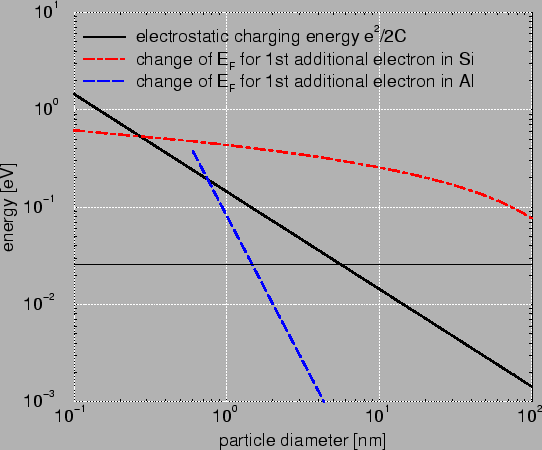Next: 2.1.2 Quantum Confinement Energies Up: 2.1 Characteristic Energies Previous: 2.1 Characteristic Energies

Christoph Wasshuber